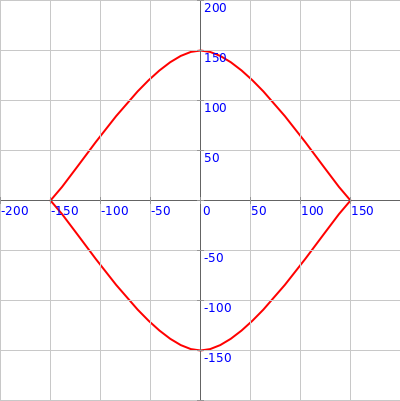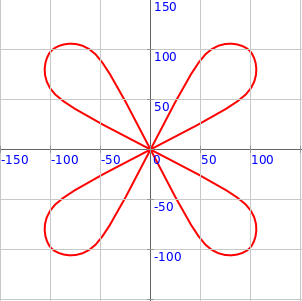## Using vertex-shape Pictures to draw shapes

This activity has the following desired goals:

• Learning to draw picture based shapes using vertices at (r, theta) cordinates (A, M).
• Using the above ideas to make interesting curved figures (M, T).

### Step 1

Type in the following code and run it:

``````clear()
showAxes()
showGrid()

val pic = Picture.fromVertexShape { s =>
import s._
beginShape()
curveVertexRt(150, 0)
curveVertexRt(150, 0)
curveVertexRt(150, 90)
curveVertexRt(150, 180)
curveVertexRt(150, 180)
endShape()
}

draw(pic)
``````

Q1a. Read through the code above and try to understand what it does. What does the above code do? How does it do it?

Q1b. How is the above code different from the very similar code in `Step 2` of the previous lesson?

### Explanation

Whatever figures you can make with the `beginShape()`, `vertex(x, y)`, `vertexRt(r, theta)`, `curveVertex(x, y)`, `curveVertexRt(r, theta)`, and `endShape()` turtle commands - can also be made using vertex-shape Pictures.

Function Description:

A vertex-shape Picture is created using the following function:

``````Picture.fromVertexShape { s =>
import s._
// your code with beginShape, vertex, curveVertex, endShape - goes here
}
``````

Inside the function, the shape being constructed is available as a parameter (called `s` above). You can call the `beginShape()` etc. commands on this `s` as usual for objects - `s.beginShape()` etc. You can also just `import s._` and then call `beginShape()` etc without putting the `s.` in front.

### Exercise

1 Write a program to make the following figure using first one and then two different vertex-shape Pictures:2 Write a program to make the following figure using first one and then four different vertex-shape Pictures: